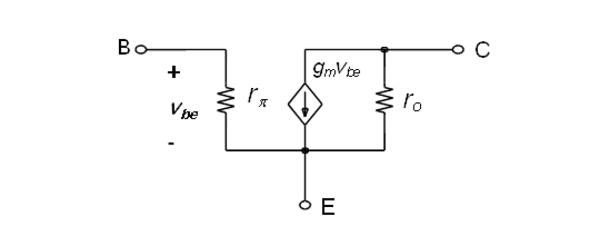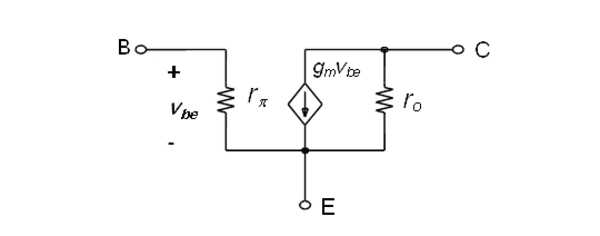# Help Understanding Current Gain Of A BJT NPN Transistor

I need to know if my formula's are correct for current gain. Sorry for shakey drawing.
I know beta would change the dc values slightly but for my prof. it's close enough.

Beta AC = 70.DC Analysis
VB = R1R2/R1+R2
VE = VB - VBE
IE = VE/RE

AC Analysis
r'e = 25mv/IE
Req = R1 || R2
Rout = RC || RL

Voltage Gain
Av = Rout/ r'e
Vout = Av * Vin

Current Gain
Ai = Iout/Iin
Iin= Vin/Rin(total)
Rin(total) = Req || Beta AC(Rin(base))
Rin(base) = Beta AC * r'e
Iout = Ic + Il
Ic = Beta AC * Iin
Il = Vout/ RL

Bolded is what I am unsure off.
I know current out is the combination of current through the collector resistor and the load resistor since AC see's DC source as a ground. So, does that mean the current in multiplied by beta is equal to the current through the collector?

Last edited by a moderator:

NascentOxygen
Staff Emeritus
Iout should be synonomous with IRL

As always, Ic = β.Ib

Right off the bat, V_b is fixed by a voltage divider consisting of R_1 and R_2. In other words, V_b is the voltage drop across R_2 only. If you combine them in parallel then what you obtain is Thevenin equivalent resistance that you would place along with Thevenin voltage which is, in turn, equal to the voltage drop across R_2.

Right off the bat, V_b is fixed by a voltage divider consisting of R_1 and R_2. In other words, V_b is the voltage drop across R_2 only. If you combine them in parallel then what you obtain is Thevenin equivalent resistance that you would place along with Thevenin voltage which is, in turn, equal to the voltage drop across R_2.

Yes. My mistake.

Iout should be synonomous with IRL

As always, Ic = β.Ib

So, if I am following correctly (which I am not sure I am), the current at the base multiplied by the beta AC is equal to the current at the collector.
So in my formulas is the current at the base Iin? So is the current at the base Iin = Vin/Rin(total)? And then to find IC, you multiply Iin by Beta AC?

NascentOxygen
Staff Emeritus
Iin is usually the current from the signal source, and comprises two components: the signal current into the base plus the signal current that goes to ground through the base biasing resistors. It is only the current into the base which gets amplified by beta.

Iin is usually the current from the signal source, and comprises two components: the signal current into the base plus the signal current that goes to ground through the base biasing resistors. It is only the current into the base which gets amplified by beta.

I am totally confused then on how to calculate current gain.
I understand that it's Iout/Iin.
I understand how to calculate Iin, but not Iout.

NascentOxygen
Staff Emeritus
Iout is that portion of collector current that goes through the load, when analysing the small signal transistor model.

Iout is that portion of collector current that goes through the load, when analysing the small signal transistor model.

Isn't that Il?
Isn't Iout the combination of the current through the collector and the load? Iout = Ic + Il?

NascentOxygen
Staff Emeritus
Isn't that Il?
Isn't Iout the combination of the current through the collector and the load? Iout = Ic + Il?
You're surely not trying to work through this exercise without sketching and labelling the circuit involving the transistor's small signal model, ARE YOU?!

The general expression for the current gain is Iout/Iin, where Iout is the current in the load, the load here being RL. The fact that you are using the terms Iout and Il for different currents when they normally are two names for the same current is just confusing.

The collector current is β.Ib and some of it goes through Rc and the remainder goes through RL. The small signal model shows this. You must always draw the small signal equivalent circuit to help with the AC analysis.

You're surely not trying to work through this exercise without sketching and labelling the circuit involving the transistor's small signal model, ARE YOU?!

The general expression for the current gain is Iout/Iin, where Iout is the current in the load, the load here being RL. The fact that you are using the terms Iout and Il for different currents when they normally are two names for the same current is just confusing.

The collector current is β.Ib and some of it goes through Rc and the remainder goes through RL. The small signal model shows this. You must always draw the small signal equivalent circuit to help with the AC analysis.r'e = 22.7Ω
Rin(base) = 70 * 22.7Ω = 1.59 kΩ
Rin(total) = 14.7 kΩ || 1.59 k ohm = 1.43 kΩ
Iin = 100mv/1.43kΩ = 69.9 μA

Ai = ? / 69.9μA

and now Im lost.

NascentOxygen
Staff Emeritus
The simplified AC model of a BJT is known as the hybrid-Pi model.rπ is what you know as β.re
gm.vbe is equivalent to β.ib
ro is ~100kΩ and can be ignored

The simplified AC model of a BJT is known as the hybrid-Pi model.rπ is what you know as β.re
gm.vbe is equivalent to β.ib
ro is ~100kΩ and can be ignored
Or the way I drew it. Still no closer to understanding how to calculate Iout though.

NascentOxygen
Staff Emeritus
For your 100mV input, what will be ib? This allows you to calculate ic.

For your 100mV input, what will be ib? This allows you to calculate ic.

ib = Rin(total)/Rin(base)?
Is that correct?

NascentOxygen
Staff Emeritus